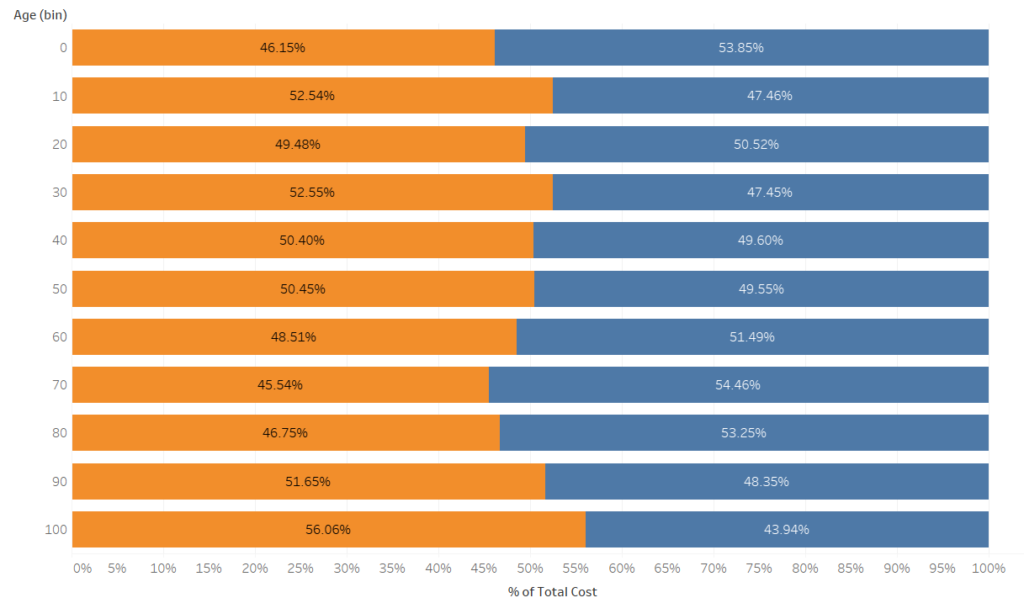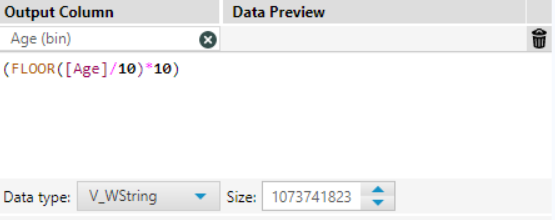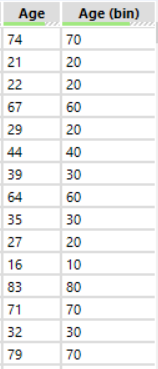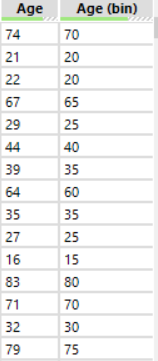## Recreating Tableau's bins in Alteryx

by Nick Jastrzebski

A few weeks ago, Andy decided to test our understanding of table calculations in Tableau by having us recreate them in Alteryx. One of the exercises he set for us was to find the percent of total of each gender across a number of ages binned into 10 year increments for a set of animals (courtesy of the truly fantastic website Mockaroo).The ages are divided up into 11 bins, one for each row in this percent of total chart.

Since the challenge was to recreate this set of data in Alteryx, we needed a way to put the ages into bins. Our first attempt was to use the tile or multi-field binning tool. This worked nicely when selecting ten bins, which would give us 0-90. However, Tableau generates 11 bins, from 0 to 100.

When trying to set 11 bins, this didn’t quite generate what we were looking for either as number weren’t split into tens brackets, so we resolved to do things ourselves!

In order to change each number into the tens category it should belong, I created the following calculation:

FLOOR(([Field to be binned]/10))*10Formula tool configuration in Alteryx

The FLOOR function forces the number to be rounded down, so by dividing the age by ten, 88 would become 8.8. Rounded down, this then becomes 8 and then multiplied by 10 again gives 80, which is the bin we want a number from 80-89 to fall into.

This also gives us the 11 bins we need as per Tableau’s bin!The ages are divided up nicely into 11 bins based on the range of 10 they fall into, from 0-100!

A voila!

For bins of varying sizes, you would just need to divide and multiply by whatever the desired bin size is. For instance, a bin size of 5 would be:

FLOOR(([Field to be binned]/5))*5

This would result in the bins looking like so:Bins set to increments of 5

This method will also work for manually creating bins in Tableau using a calculated field, rather than the automatic method of creating bins, if that should ever be unavailable to you!Nick Jastrzebski

Fri 30 Nov 2018

Fri 02 Nov 2018

Thu 01 Nov 2018